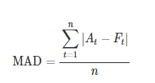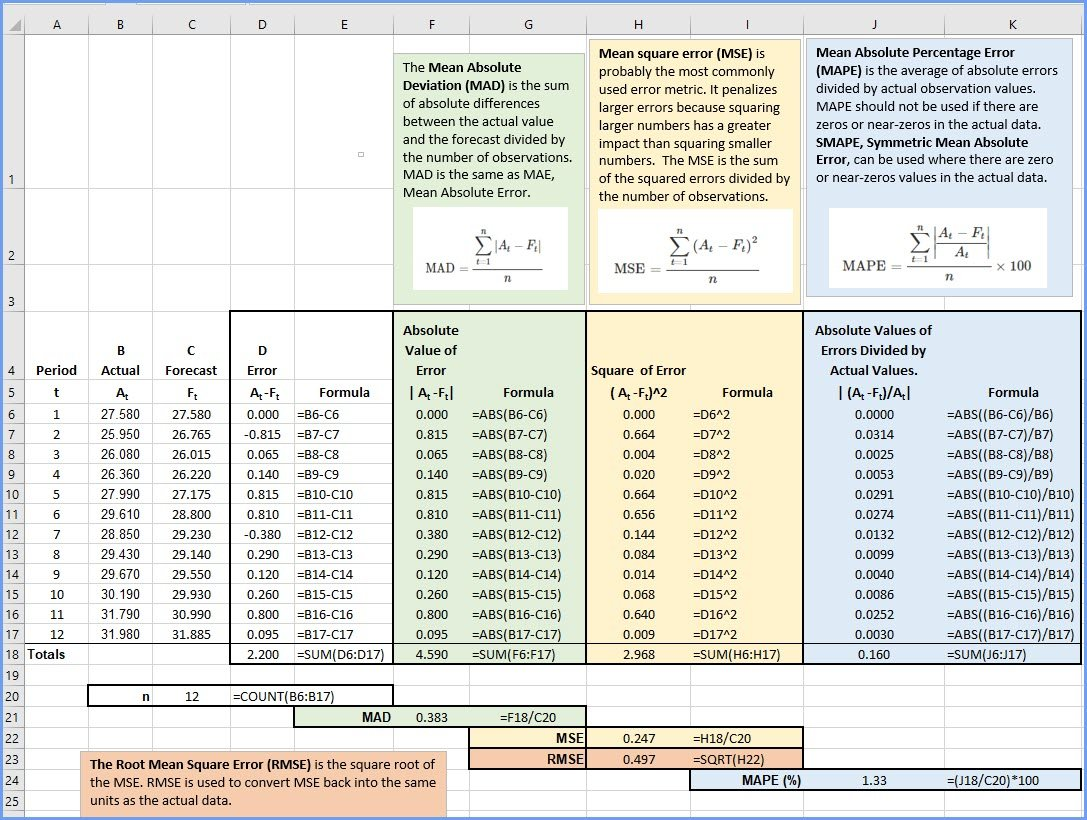# Use Excel to Calculate MAD, MSE, RMSE & MAPETo optimize your forecast, whether moving average, exponential smoothing or another form of a forecast, you need to calculate and evaluate MAD, MSE, RMSE, and MAPE. With Excel 2016 or later, this is easy to do.

The Mean Absolute Deviation (MAD) is the sum of absolute differences between the actual value and the forecast divided by the number of observations. MAD is the same as MAE, Mean Absolute Error.Mean square error (MSE) is probably the most commonly used error metric. It penalizes larger errors because squaring larger numbers has a greater impact than squaring smaller numbers.  The MSE is the sum of the squared errors divided by the number of observations.The Root Mean Square Error (RMSE) is the square root of the MSE. RMSE is used to convert MSE back into the same units as the actual data.Mean Absolute Percentage Error (MAPE) is the average of absolute errors divided by actual observation values. MAPE should not be used if there are zeros or near-zeros in the actual data. SMAPE, Symmetric Mean Absolute Percent Error, can be used where there are zero or near-zeros values in the actual data.Symmetric Mean Absolute Percent Error (SMAPE) is an alternative to Mean Absolute Percent Error (MAPE) when there are zero or near-zero values in your actual observations. SMAPE self-limits to an error rate of 200%, reducing the influence of zero or near-zeros observations. SMAPE is the forecast minus actuals divided by the sum of forecasts and actuals as expressed in this formula:View the video explaining the workbook here: https://youtu.be/H_N5_vxs8Tg

Video explaining how to interpre the error metrics here:  https://youtu.be/vxtxrcdmqbw

### 11 thoughts on “Use Excel to Calculate MAD, MSE, RMSE & MAPE”

1.Thanks for the help, professor!

2.Amazing!

3.Thank you

1.Thank you for the positive feedback!

4.how did you get the forecast

1.This is just an example of a typical forecast. You can develop your forecast using many different methods: simple regression or one of the many time-series methods. These error metrics can be applied to a variety of numerical forecasts.

5.Thank you!!

6.God bless you richly, please I need such on AIC, BIC and loglikelihood. Thanks

7.Thank you sir, this really good, I’ve been trying to get MAPE on every way in excel but didn’t work until i got this, thank you.

8.Thank you, sir, I cannot download the excel file, is it still available? Please, could you provide me with it?

1.